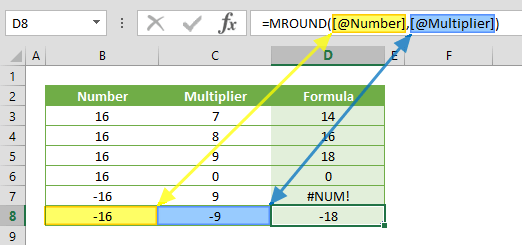The MROUND function rounds a number to the nearest multiple of a specified number. In this guide, we’re going to show you how to use the MROUND function and also throw in some tips and error handling methods.

• All versions

# Syntax

MROUND(number,multiple)

# Arguments

 Number The number to be rounded. Multiple The multiple to which you want to round number.

Examples

Firstly a reminder: Named ranges are added to ease of reading formulas.

## Example 1

=MROUND(16,9)
formula returns 18. The 9 has 2 multiples near to 16, which are 9 and 18. Because the 18 is the nearest, the formula returns 18.## Example 2

=MROUND(-16,-9)
formula returns -18 according to similar logic with Example 1. On the other hand, if the number is negative, choose a negative number for the multiplier as well.# Tips

• Use the ROUND for standard rounding.
• Use the ROUNDUP to always round up.
• Use the ROUNDDOWN to always round down.

# Issues

## #NUM!

If the number and multiplier has opposite signs (+/-), the #NUM! error is returned.